It is the force of attraction exerted by earth towards its centre on a body lying on or near the surface of the earth. Gravity is the measure of the weight of the body.

The weight of a body of mass m = mass X acceleration due to gravity = mg.

The unit of weight of a body will be the same as those of force.

### Acceleration due to gravity (g):

It is defined as the acceleration set up in a body while falling freely under the effect of gravity alone. It is a vector quantity. The value of g changes with height, depth, rotation of earth the value of g is zero at the centre of the earth. The value of g on the surface of the earth is 9.8ms-2.

The acceleration due to gravity (g) is related with gravitational constant (G) by the relation,

where M and R are the mass and radius of the earth.

Explanation: The force on the mass m at P has a magnitude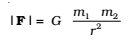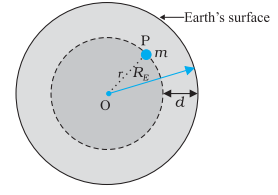The mass m is a mine located at a depth d below the surface of the Earth of mass ME and radius RE. We treat the Earth to be spherically symmetric.

We assume that the entire earth is of uniform density and hence its mass iswhere ME is the mass of the earth RE is its radius and ρ is the density. On the other hand, the mass of the sphere Mr of radius r is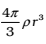and hence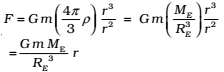If the mass m is situated on the surface of earth, then r = RE and the gravitational force on it is,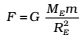The acceleration experienced by the mass m, which is usually denoted by the symbol g is related to F by Newton’s 2nd law by relation F = mg. Thus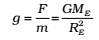• Variation of acceleration due to gravity
• Effect of altitude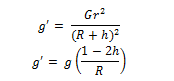The first is valid when h is comparable with R and the second relation is valid when h << R.

The value of g decreases with increase in h.

• Effect of depth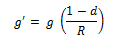The acceleration due to gravity decreases with increase in depth d and becomes zero at the centre of the earth.

• Effect of rotation of earth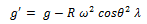The acceleration due to gravity on equator decreases on account of rotation of the earth and increase with the increase in latitude of a place.